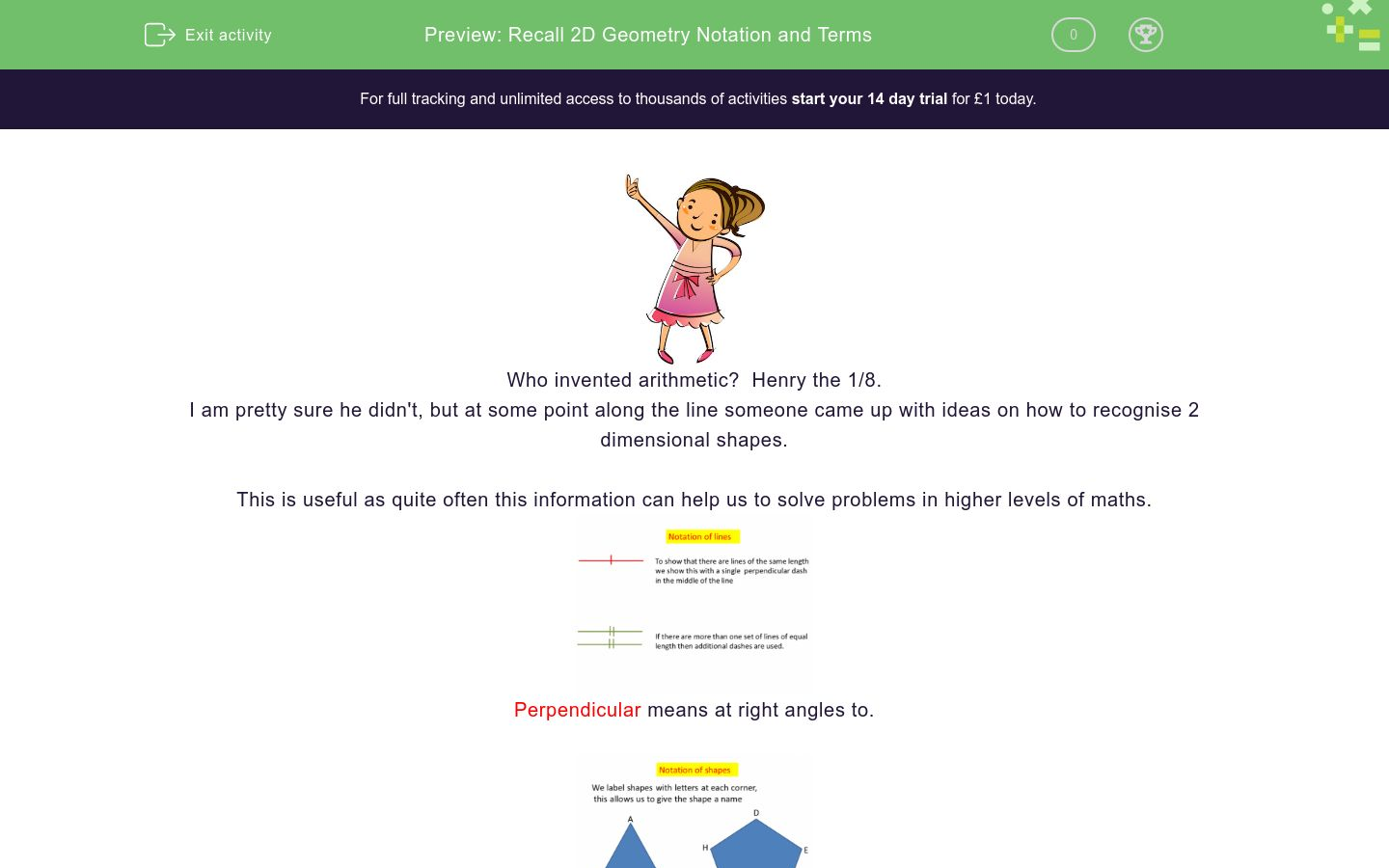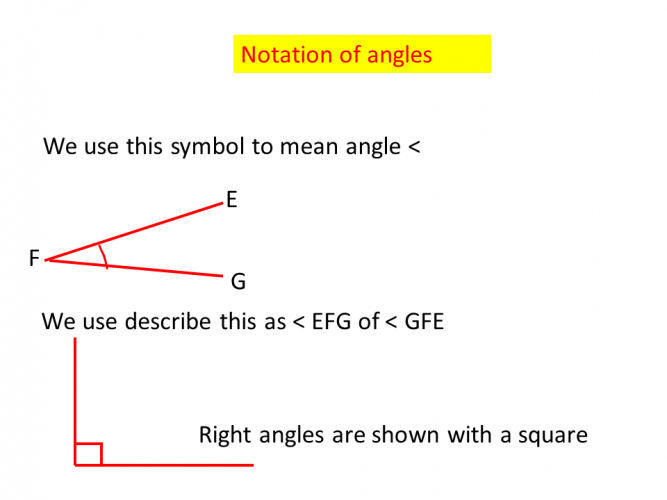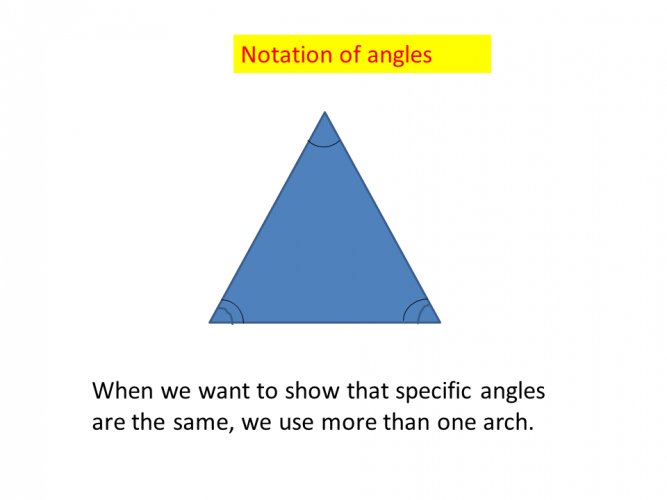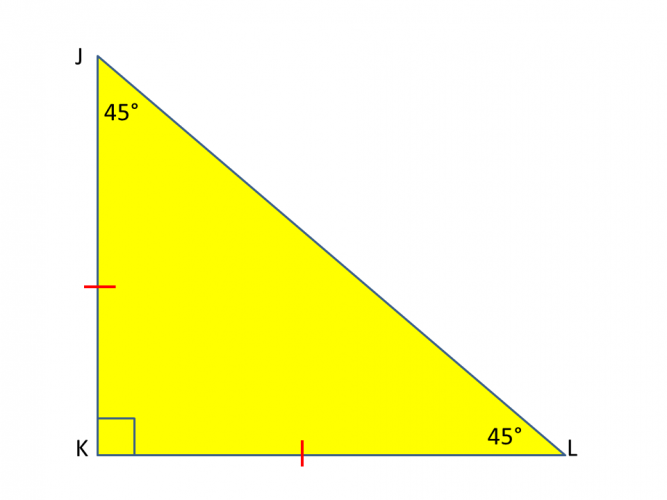# Recall 2D Geometry Notation and Terms

In this worksheet, students will learn the correct the notation for 2D shapes.Key stage:  KS 4

GCSE Subjects:   Maths

GCSE Boards:   AQA, Eduqas, Pearson Edexcel, OCR

Curriculum topic:   Geometry and Measures, Basic Geometry

Curriculum subtopic:   Properties and Constructions, Conventions, Notations and Terms

Difficulty level:### QUESTION 1 of 10Who invented arithmetic?  Henry the 1/8.

I am pretty sure he didn't, but at some point along the line someone came up with ideas on how to recognise 2 dimensional shapes.

This is useful as quite often this information can help us to solve problems in higher levels of maths.Perpendicular means at right angles to.Over to youWhich statements are true?

< ABC = 48°

< CAB= 48°

< ACB = 48°

< BCA= 48°

Match the following

## Column B

Perpendicular means
Give a triangle a name
< symbol identifies
Lines that never meet.
Parallel means
At right angles to
ABC and DEF
An angle1 2 3 4 How many sets of lines are the same length? How many sets of parallel lines are there?True or False. < EGF = 52°What does this notation tell you?

One pair of lines the same length

There are two sets of parallel lines

Two pairs of lines are the same

There is one set of parallel linesWhat type of triangle is this?What type of triangle is this?

 Isosceles Scalene Equilateral The triangle isThis is the shape of a kite.

What marking would you place on it.

| and | with || and ||

) and ) with )) and ))

) and )

| and |True False The triangle is labelled correctly

Match up the following statements

## Column B

A shape with one set of parallel sides is
a parallelogram
A triangle with a different number of arches at ea...
a kite
A shape with two sets of parallel sides and two se...
scalene
A shape with two sets of line lengths the same and...
a trapezium
• Question 1Which statements are true?

< ACB = 48°
< BCA= 48°
EDDIE SAYS
Shapes are 'read' in alphabetical order. Have you noticed the middle letter is the angle identified.
• Question 2

Match the following

## Column B

Perpendicular means
At right angles to
< symbol identifies
An angle
Parallel means
Lines that never meet.
ABC and DEF
Give a triangle a name
EDDIE SAYS
The important thing here is to know the definitions. These are used a lot in shape work. Once you understand it all, it makes your higher level work a little easier.
• Question 31 2 3 4 How many sets of lines are the same length? How many sets of parallel lines are there?
EDDIE SAYS
Were you able to separate the lines out? From the markings you can see that | and | show one set of equal lines. || and || show the other set of equal lines. > and > show one set of parallel lines and >> and >> show the other set of parallel lines.
• Question 4True or False. < EGF = 52°

False
EDDIE SAYS
Remember how to read the triangle. EGF shows no angle so the answer is false.
• Question 5What does this notation tell you?

There is one set of parallel lines
EDDIE SAYS
As there is only one set of notations this makes life easier. There is only one thing being pointed out and that is that the set of lines identified will never meet so therefore they are parallel.
• Question 6What type of triangle is this?

Equilateral
EDDIE SAYS
All the markings on the lines and in the angles are the same which tells us they are equal. Therefore it is an equilateral triangle.
• Question 7What type of triangle is this?

 Isosceles Scalene Equilateral The triangle is
EDDIE SAYS
Our notation tells us that the two angles at the bottom are the same as they both have two arches. The two slanted sides are also marked with a single line which tells us they are the same length. These properties are found in an isosceles triangle.
• Question 8This is the shape of a kite.

What marking would you place on it.

| and | with || and ||
) and )
EDDIE SAYS
Are you flying high with this one? The two longer sides are the same length, the two shorter sides are the same length. Where a long line meets a short line the angles are the same.
• Question 9True False The triangle is labelled correctly
EDDIE SAYS
The angles are marked for us. 45 and 45 are base angles to the two sides that are marked as the same length. The angles 90 + 45 + 45 add up to 180° so therefore this is an isosceles triangle.
• Question 10

Match up the following statements

## Column B

A shape with one set of parallel ...
a trapezium
A triangle with a different numbe...
scalene
A shape with two sets of parallel...
a parallelogram
A shape with two sets of line len...
a kite
EDDIE SAYS
Hopefully you can see the importance of learning the notation not just in diagram form, but also in written form. The notation and terms may be presented in either way.
---- OR ----

Sign up for a £1 trial so you can track and measure your child's progress on this activity.

### What is EdPlace?

We're your National Curriculum aligned online education content provider helping each child succeed in English, maths and science from year 1 to GCSE. With an EdPlace account you’ll be able to track and measure progress, helping each child achieve their best. We build confidence and attainment by personalising each child’s learning at a level that suits them.

Get started